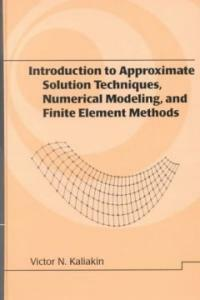> Detail View

# Detail View## Introduction to approximate solution techniques, numerical modeling, and finite element methods (Loan 2 times)

Material type
단행본
Personal Author
Kaliakin, Victor N.
Title Statement
Introduction to approximate solution techniques, numerical modeling, and finite element methods / Victor N. Kaliakin.
Publication, Distribution, etc
New York :   Marcel Dekker,   c2002.
Physical Medium
xvi, 674 p. : ill. ; 24 cm.
Series Statement
Civil and environmental engineering ;9
ISBN
082470679X (hbk. : alk. paper) 9780824706791 (hbk. : alk. paper)
Content Notes
1. Governing Equations and Their Approximate Solution -- 2. Computer Storage and Manipulation of Numbers -- 3. The Finite Difference Method -- 4. The Method of Weighted Residuals -- 5. Variational Methods -- 6. Introduction to the Finite Element Method -- 7. Development of Finite Element Equations -- 8. Steps in Performing Finite Element Analyses -- 9. Element Interpolation Functions -- 10. Element Mapping -- 11. Finite Element Analysis of Scalar Field Problems -- 12. Finite Element Analysis in Linear Elastostatics -- 13. Implementation, Modeling, and Related Issues -- A. Mathematical Potpourri -- B. Some Notes on Heat Flow -- C. Local and Natural Coordinate Systems -- D. The Patch Test -- E. Solution of Linear Systems of Equations -- F. Notes on Integration of Finite Elements.
Bibliography, Etc. Note
Includes bibliographical references (p. 613-663) and index.
Finite element method.
 000 02050camuu2200349 a 4500 001 000045365568 005 20070626105705 008 010724s2002 nyua b 001 0 eng 010 ▼a 2001047122 020 ▼a 082470679X (hbk. : alk. paper) 020 ▼a 9780824706791 (hbk. : alk. paper) 024 3 1 ▼a 9780824706791 035 ▼a (OCoLC)ocm47696342 035 ▼a (OCoLC)47696342 035 ▼a (KERIS)REF000006500253 040 ▼a DLC ▼c DLC ▼d C#P ▼d KKU ▼d OrLoB-B ▼d 211009 042 ▼a pcc 050 0 0 ▼a TA347.F5 ▼b K35 2002 082 0 0 ▼a 620/.001/51535 ▼2 21 082 0 4 ▼a 620.001/51825 ▼2 22 090 ▼a 620.00151825 ▼b K14i 100 1 ▼a Kaliakin, Victor N. 245 1 0 ▼a Introduction to approximate solution techniques, numerical modeling, and finite element methods / ▼c Victor N. Kaliakin. 260 ▼a New York : ▼b Marcel Dekker, ▼c c2002. 300 ▼a xvi, 674 p. : ▼b ill. ; ▼c 24 cm. 440 0 ▼a Civil and environmental engineering ; ▼v 9 504 ▼a Includes bibliographical references (p. 613-663) and index. 505 0 ▼a 1. Governing Equations and Their Approximate Solution -- 2. Computer Storage and Manipulation of Numbers -- 3. The Finite Difference Method -- 4. The Method of Weighted Residuals -- 5. Variational Methods -- 6. Introduction to the Finite Element Method -- 7. Development of Finite Element Equations -- 8. Steps in Performing Finite Element Analyses -- 9. Element Interpolation Functions -- 10. Element Mapping -- 11. Finite Element Analysis of Scalar Field Problems -- 12. Finite Element Analysis in Linear Elastostatics -- 13. Implementation, Modeling, and Related Issues -- A. Mathematical Potpourri -- B. Some Notes on Heat Flow -- C. Local and Natural Coordinate Systems -- D. The Patch Test -- E. Solution of Linear Systems of Equations -- F. Notes on Integration of Finite Elements. 650 0 ▼a Finite element method. 945 ▼a KINS

### Holdings Information

No. Location Call Number Accession No. Availability Due Date Make a Reservation Service
No. 1 Location Call Number 620.00151825 K14i Accession No. 121148681 Availability Available Due Date Make a Reservation Service

### Contents information

`Governing equations and their approximate solution; computer storage and manipulation of numbers; the finite difference method; the method of weighted residuals; variational methods; introduction to the finite element method; development of finite element equations; steps in performing finite element analysis; element interpolation functions; element mapping; finite element analysis of scalar field problems; finite element analysis in linear elastostatics; implementation, modelling, and related issues. Appendices: mathematical potpourri; matrices and linear algebra; some notes on heat flow; local and natural coordinate systems; the patch test; solution of linear systems of equations; notes on integration of finite elements.`

Information Provided By: :### New Arrivals Books in Related Fields

#### 공학수학 문제풀이

Zill, Dennis G. (2022)

#### Design, fabrication and characterization of multifunctional nanomaterials

Thomas, Sabu (2021)

#### 통계적 품질관리

Montgomery, Douglas C. (2022)

#### 재료과학과 공학

Callister, William D. (2021)

#### Multiscale modeling approaches for composites

Chatzigeorgiou, George (2022)

장경욱 (2022)

#### Current research in neuroadaptive technology

Fairclough, Stephen H (2022)

김도영 (2022)

#### 재료공학개론

Shackelford, James F. (2022)

이지웅 (2021)

이정원 (2022)

#### 공학수학

Zill, Dennis G. (2022)

유봉조 (2022)

송철기 (2022)

이시중 (2022)

#### (Beer의) 재료역학

Beer, Ferdinand Pierre (2022)